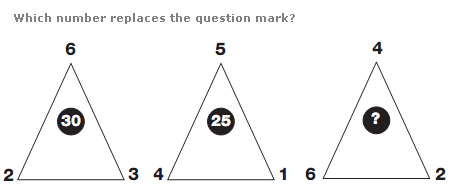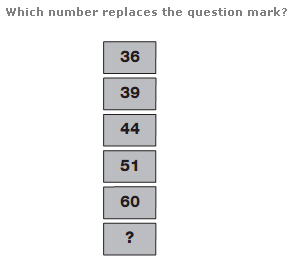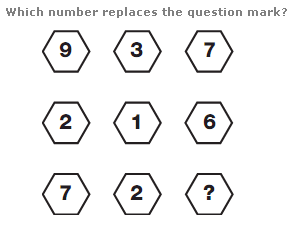# Puzzles - Number puzzles

### Exercise :: Number puzzlesAnswer : 32 Explanation : In each triangle, add together the lower 2 digits, and multiply this by the top digit, to give the value written in the centre of the triangle.Answer : 71 Explanation : As you move down, numbers follow the sequence of Square Numbers, from 36 to 121, subtracting 10 for the second number, 20 for the next, then 30 etc.Answer : 1 Explanation : Working in columns, start with the top number, and subtract the number in the centre, to give the result in the bottom row.